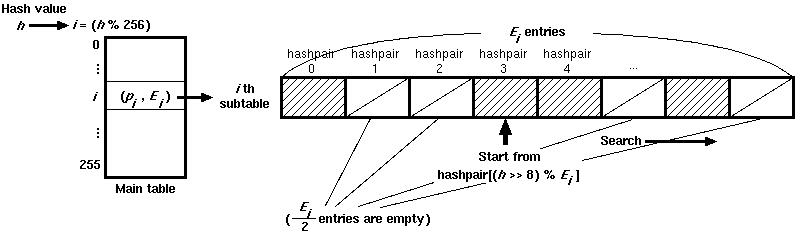## Constant Database (cdb) Internals

Constant Database, known as cdb, is an elegant data structure proposed by D. J. Bernstein. It is suitable for looking up static data which is associated with arbitrary byte sequences (usually strings). Although DJB already explained this in his cdb page, it is not easy to implement this because his document lacks some important information such as the number of each subtable. Here I tried to illustrate it for those who try to develop or extend a cdb implementation.

### Overall Structure

A cdb has a number of records which has a key and a datum, each of which can be in variable length (up to 4GBytes). The file format is designed for locating a record with as few seeks as possible. Although a cdb itself seems to have a flat list of key and datum pairs, in fact it has 2-level hierarchical tables to lookup. A cdb file consists of roughly three parts. Actual data which consist of an arbitrary number of key/datum pairs are sandwiched between two lookup tables. The first lookup table has always fixed size (2,048 bytes). The second lookup table is divided into at most 256 subtables, each of which has a variable number of entries, and is accessed from the pointer stored in the first table. (Fig. 1)Fig. 1: Cdb File Structure

### Table Lookup

Each key/value pair is referred to by a small data structure which is stored in the second table. Here we call it a "hashpair" for convenience.

1. Given a key, a 32-bit hash value h is calculated from the key by a certain function:
2. A hashpair which has the hash value h is located. This step divides into two phases (Fig. 2):
1. The (h % 256) [the least 8 significant bits of h] th subtable is located from the first lookup table. ith subtable contains Ei hashpairs. The first lookup table has 256 pairs of the pointer pi and the number of entries Ei for each subtable. Ei can be zero if the subtable is not existent.
2. The subtable entries are scanned to find the hashpair. The search starts from ((h >> 8) % Ei) th hashpair. If it reaches the end of the subtables, the search goes back at the beginning of the table. To facilitate searching, exactly Ei/2 entries are kept empty (where the pointer is NULL) to detect nonexistent keys. If it reaches an empty slot, that means there is no corresponding data in this file.
3. The actual key/datum pair is accessed through the pointer in the hashpair.
4. If the key is correct, the corresponding datum is retrieved. Otherwise, the next hashpair is tried. This process continues until either all hashpairs in the subtable are tried or an empty hashpair is found.Fig. 2: Hashpair Lookups

(TODO)

### Performance

I measured the lookup time and file size for randomly created records. The test program is dbmperf.c. You need gdbm and Samba source to build. Here is the result. Times are in seconds. File sizes are in bytes.

#### Lookup time (Pentium III, 1GHz)

# of records 10,000 100,000 1,000,000
gdbm0.070 0.862 9.141
tdb 0.035 0.358 3.904
cdb 0.028 0.288 3.068

#### File size

# of records 10,000 100,000 1,000,000
gdbm 1,359,459 14,316,032 139,738,235
tdb 1,081,344 10,764,288 107,667,456
cdb 986,108 9,798,122 98,005,628

Σ Ei = 2 * (the total number of records)

### Sample Implementation

Here is a sample implementation of cdb in Python. The plain text version is here.